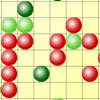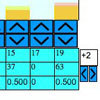You may also likeSpinners

How do scores on dice and factors of polynomials relate to each other?Epidemic Modelling

Use the computer to model an epidemic. Try out public health policies to control the spread of the epidemic, to minimise the number of sick days and deaths.Distribution Maker

This tool allows you to create custom-specified random numbers, such as the total on three dice.

Random Squares

Age 16 to 18 Challenge Level:

This is a very open ended problem with no 'full solution' as such, but we can still make some inroad into analysing the situation using a mixture of experimentation, calculation and extrapolation.

Josh and Philip from Cheshire looked at smaller grids and described them using solid lines of colour

The large squares looked complicated so we first looked at the 2 by 2 squares. We decided that the best way to describe them would be to say a) square one colour only b) 3 squares one colour and c) Two squares of each colour.

For the 3 by 3 squares we looked at the total number of solid lines of colour going right across the grid (including diagonals). Possibilities were 0, 1, 2, 3, 4, 8.

Looking at complete lines for the 4 x 4 case seemed to work sensibly, but for larger squares we only got solid lines across the grid when we changed the probability to make one of the colours a lot more likely.

PK looked at the chance of a chequerboard pattern happening randomly. PK said

For a chess board pattern the top left square can be any colour and then the rest of the squares must be the right colour to make the pattern. So, an n by n square would need n x n - 1 squares to randomly be the right colour. For even probability, the chance would be 1 in 2 to the power (n x n -1). Look at this table to see these
 Size of square Number of squares to get right Chance 2 x 2 3 1 in 8 3 x 3 8 1 in 256 4 x 4 15 1 in 32768 5 x 5 24 1 in16777216

The chance of the 10 by 10 grid would be 1 in 2 to the power 99!
So, even with a 3 x 3 grid the chance is very small. I would not believe anyone on a larger grid unless hundreds or thousands of people did the experiment. I think that you are most likely to get a chess board with 0.5 chance of red. I couldn't work this out so I did an experiment. Using the program on the webpage I looked at 2 by 2 with chance of red 0.9 and only got about 3 chequerboards each time, so it does seem less likely.

Fifi from Glenarm College then sent in these interesting observations. We loved the way Fifi approached the problem, looking at concrete cases and making some general predictions and observations. Very well done!

The large squares looked complicated so we first looked at the 2 by 2 squares. We decided that the best way to describe them would be to say a) square one colour only b) 3 squares one colour and c) Two squares of each colour. For the 3 by 3 squares we looked at the total number of solid lines of colour going right across the grid (including diagonals). Possibilities were 0, 1, 2, 3, 4, 8. Looking at complete lines for the 4 x 4 case seemed to work sensibly, but for larger squares we only got solid lines across the grid when we changed the probability to make one of the colours a lot more likely.

PK looked at the chance of a chequerboard pattern happening randomly. PK said For a chess board pattern the top left square can be any colour and then the rest of the squares must be the right colour to make the pattern. So, an n by n square would need n x n - 1 squares to randomly be the right colour. For even probability, the chance would be 1 in 2 to the power (n x n -1). Look at this table to see these:

 Size of grid Number of squares to get right Chance 2 x 2 3 1 in 8 3 x 3 8 1 in 256 4 x 4 15 1 in 32768 5 x 5 24 1 in16777216

The chance of the 10 x 10 grid would be 1 in 2 to the power 99, which is absolutely tiny. So, even with a 3 x 3 grid the chance is very small. I would not believe anyone on a larger grid unless hundreds or thousands of people did the experiment. I think that you are most likely to get a chess board with 0.5 chance of red. I couldn't work this out so I did an experiment. Using the program on the webpage I looked at 2 by 2 with chance of red 0.9 and only got about 3 chequerboards each time, so it does seem less likely.